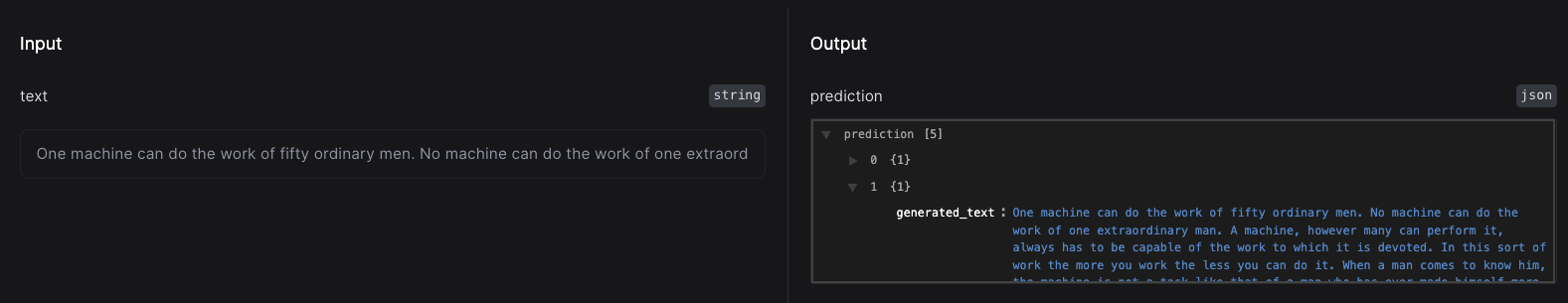You may wish to use Slai for packaging and deployment, while doing your training elsewhere. Let’s walk through a quick tutorial on how you can use pre-trained models in your Slai project.

In this example, we’ll use a pre-trained GPT-2 model from HuggingFace.

Let’s start with train.py. Simply import your pre-trained model in train.py and return the model class in train()

train.py
import transformers import pipeline, set_seet

def train():
mode = pipeline("text-generation", model="gpt-2")
set_seed(42)

return model


Now, let’s move onto handler.py. In your handler, you can define how you’d like to handle the inputs and outputs to the pre-trained model. In this example, we’ll pass a string input, and return a JSON object with generated sentences from GPT-2.

handler.py
import slai

class ModelHandler(slai.BaseModelHander):
inputs = {"text": slai.types.String()}

outputs = {
"prediction": slai.types.Json(),
}

def call(self, artifact, **inputs):
gpt2 = artifact
prediction = gpt2(inputs["text"], max_length=500, num_return_sequences=5)
return {"prediction": prediction}


Even though the model is trained, you’ll need to click Train to download the pre-trained weights into the sandbox. Once that is complete, you can test the model before deploying.

In the test panel, you’ll get an interactive test utility to send requests to the model. Let’s generate some sentences!Using the test utility